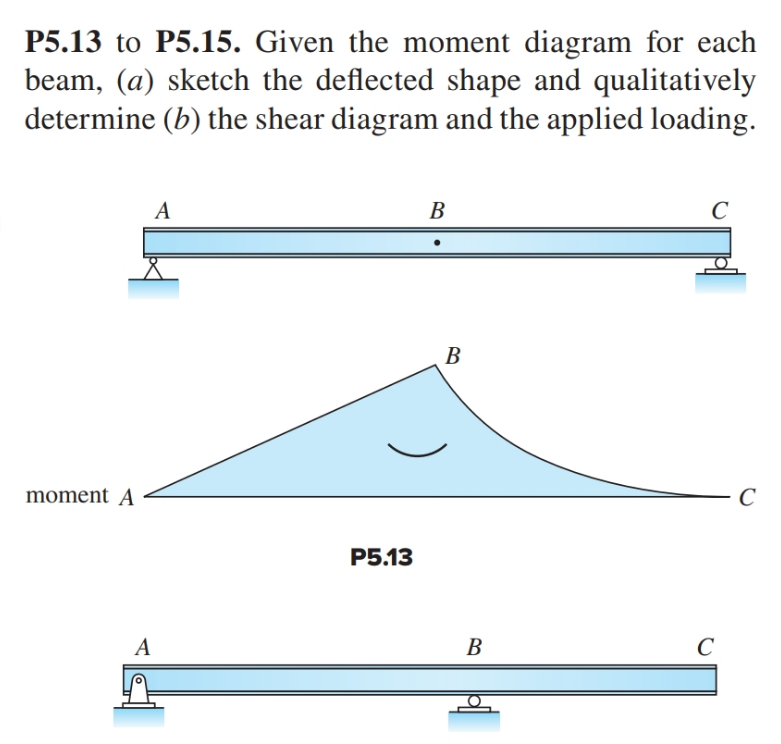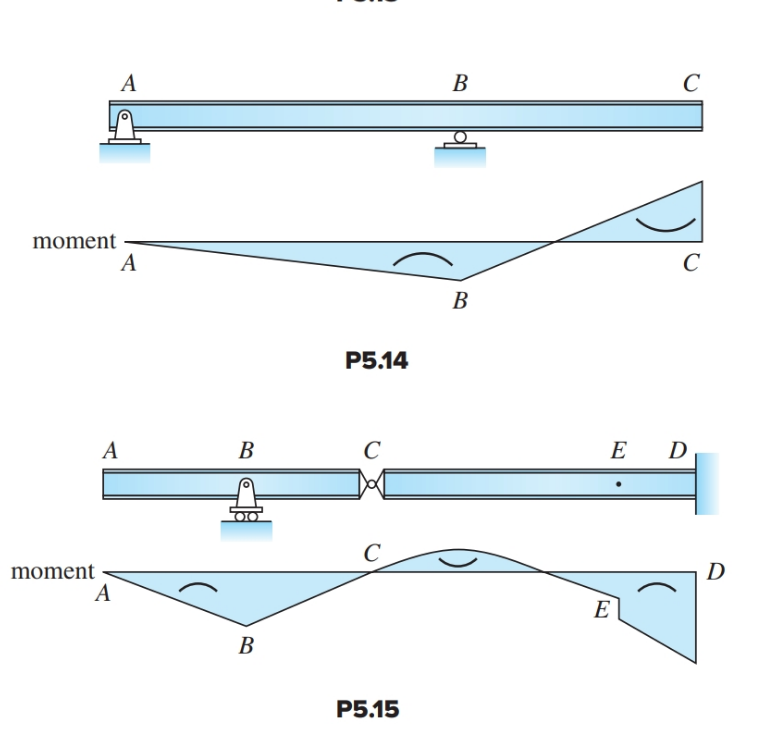# Question P5.13 to P5.15. Given the moment diagram for each beam, (a) sketch the deflected shape and qualitatively determine (b) the shear diagram and the applied loading. A B С B moment A С P5.13 A B C A B С moment A C B P5.14 A B C E D С moment A D E B P5.15JVHKTL The Asker · Civil EngineeringTranscribed Image Text: P5.13 to P5.15. Given the moment diagram for each beam, (a) sketch the deflected shape and qualitatively determine (b) the shear diagram and the applied loading. A B С B moment A С P5.13 A B C A B С moment A C B P5.14 A B C E D С moment A D E B P5.15
More
Transcribed Image Text: P5.13 to P5.15. Given the moment diagram for each beam, (a) sketch the deflected shape and qualitatively determine (b) the shear diagram and the applied loading. A B С B moment A С P5.13 A B C A B С moment A C B P5.14 A B C E D С moment A D E B P5.15